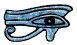# Trigonometry and the Sine and Cosine Rules

Introduction

If we are given one side of a triangle and two other pieces of information about it we can use the Sine and Cosine Rules to calculate all the other sides and angles. There are some worked Examples on the Sine and Cosine Rules on this Page and you can go straight to them if you want to, but if you try to use the Rules without understanding why you need to do the calculations in a certain order you may well end up in a mess. So this Page is intended to help you understand them.

All the angles add up to 180°. This means that a triangle can have three acute angles or two acute angles and one obtuse angle. If we look at the graph of sinθ and cosθ between 0° and 180° we can see that for each value of cosθ there is only one value of θ, and for angles between 90° and 180° cosθ is negative but for each value of sinθ there are two values of θ, one acute and one obtuse and adding up to 180°.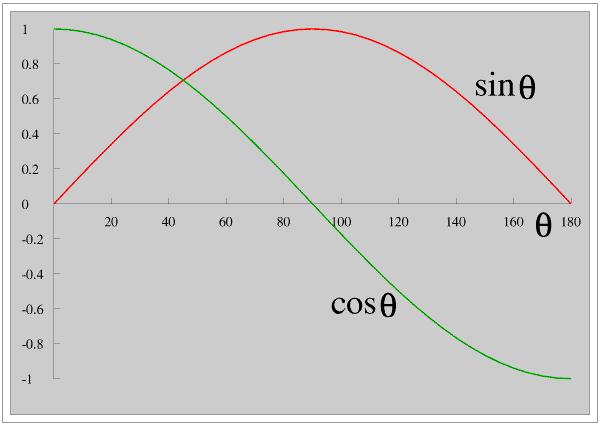This means that when you are using the Sine Rule you must try to solve the triangle in a way which allows you to know in advance whether the angle you are finding is acute or obtuse: this is not difficult provided you follow these guidelines. If you do not follow these Guidelines you will almost certainly end up with, at best just the wrong answer, at worst the wrong answer and a lot of wasted time, if the triangle you are solving contains an obtuse angle.

• All the angles in a triangle add up to 180°
• The largest angle is opposite the longest side
• A triangle must always have at least two acute angles
• If a is less than b then A is less than B so A must be acute.

# Worked Examples

Each of these Examples deals with a different set of conditions. Each Page is self-contained so you can print it off if you think this will be helpful to you.

All the lengths are in millimetres to the nearest millimetre and all the angles are in degrees to the nearest degree. In most Examples the triangle is not drawn accurately so that you really have to think about what you are doing.

## Appendices - For Advanced Readers Only

Lots of words in maths and science come from Greek or Latin words; lots of other words, particularly words beginning with al such as alkali and algebra, come from Arabic.

A shape with straight line sides is called a polygon, from the Greek for many sides, although most people only use the word for shapes with more than four sides. A pentagon has five sides, similarly hexagon, heptagon, octagon etc.

We could call a four-sided shape a tetragon from the Greek for four sides, but we usually call it a quadrilateral, from the Latin for four lines, and similarly we could call a three-sided shape a trigon, but we usually call it a triangle, from the Latin for three corners. (But we do call a triangular based pyramid a tetrahedron, from the Greek for four faces.)

If we have a triangle and we double the length of one side while keeping the angles the same we also necessarily (that is, it must happen, we cannot stop it from happening) double the length of both the other sides - the triangles are the same shape but not the same size: we say they are similar. For any triangle the ratio of the length of the sides depends only on the size of the angles, and visa versa. This is always true of all triangles, it is never true of any quadrilateral or any other polygon. For example in a square all the angles are right angles. If we keep the angles the same but double the length of one side we necessarily double the length of the opposite side but do not necessarily change the length of the other two sides (that is they do not automatically change) and so end up with a rectangle. (Advanced Readers might like to read my Page on Paper Sizes - currently under construction.)

A whole branch of mathematics is based upon this very simple idea, we call it trigonometry, from the Greek for measuring with triangles.

Trigonometry is very important and can be very exciting, but sadly the way it is often taught in schools makes it appear dull and irrelevant, which is a pity. The satnav in your car or phone or tablet or camera works because of trigonometry, see my Page on the GPS.

## Triangles and the Sine and Cosine Rules

A triangle has three sides and three angles. If we have a triangle and we are given the length of one side and any two other pieces of information we can use the Sine and Cosine Rules to find all the other sides and all the other angles. This is called solving the triangle.

If we are given the three angles but no sides we can draw lots of triangles, but they will all be similar, that is, the same shape but not the same size. We can easily find the ratio of the sides, but to solve a triangle we must always be given at least one side.

We normally label the vertices of a triangle with capital (upper case) letters, such as A, B and C - the lower case letters a, b and c on the diagram are explained later on this Page.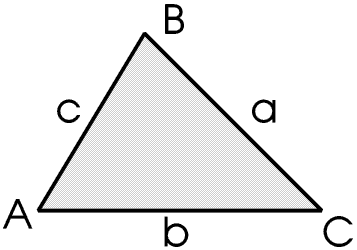The sides are AB, AC and BC - the order in which we write the letters does not matter unless they are vectors - vectors are described on another Page.

The angle at A is usually referred to as angle A unless there is more than one angle there, in which case we must refer to it as angle BAC.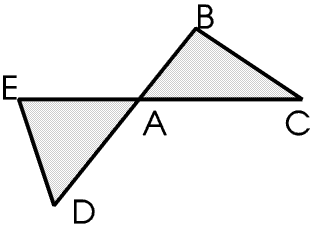Angle A is included between BA and AC, and is opposite BC. We usually use lower case letters to denote the lengths of the sides, so the length of BC, which is opposite angle A, is a, and similarly the length of AC (opposite B) is b and the length of AB (opposite C) is c.

The Cosine Rule has two forms. In an exam at or before normal school-leaving age one of them will usually be on the Formula Sheet provided with the question sheet, and it is not difficult to change the subject to get the other; the Sine Rule has only one form and is very easy to remember so you are not always given it.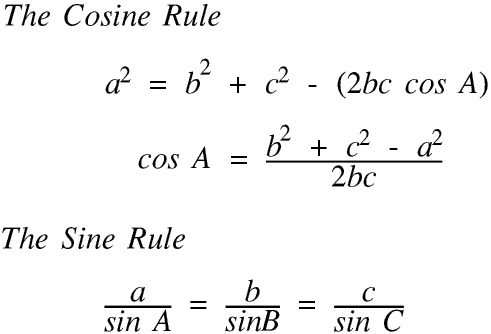The Proof of these two Rules is given in Appendix 1

In an exam question or homework exercise the vertices of the triangle may not be labelled A, B and C. Unless you are very confident, particularly with the Cosine Rule, never be afraid to redraw the triangle on a separate sheet of paper with the vertices labelled A, B and C - for reasons given later A should be the largest angle or face the longest side.

You should not try to use the Sine and Cosine Rules without a proper scientific calculator: many of the calculator programs or apps on your PC or Laptop or Tablet or Phone do not contain sine and cosine (and tangent) keys, and many of those that do not always work correctly with these Rules in every possible situation - and in any case you will probably not be allowed to use your phone or anything else with an internet connection in an exam.

Almost every calculation involving the sin or cos or sin-1 or cos-1 keys will produce an irrational answer so your calculator will store the result to an accuracy of about fifteen significant figures and display it to ten, but you will need to round it sensibly before writing the answer down; when using the result in another calculation you must always use the unrounded value. This is stored in ANS in your calculator so make certain you know how to use the ANS key. Irrational Numbers and Using the ANS Key are explained on their own Pages, and the second of these Pages also demonstrates the importance of using unrounded values in on-going calculations. It also tells you how your calculator stores earlier calculations, which is sometimes useful when using the Sine and Cosine Rules.

We can have the sines and cosines (and tangents) of any angle, not only of angles between 0° and 90° - this is more fully discussed on the Page on Cartesian and Polar Co-Ordinates. We normally use the Greek letter θ (theta) for the size of an angle. Here are the values of sin θ and cos θ for values of θ between 0° and 180°

For angles between 0° and 180° sin θ must be in the range 0 to 1 and cos θ must be in the range -1 to +1. If you find a value outside this range you have probably made a mistake so you should check your work; there is however one very special case, when you find sin θ to be between 1.00 and 1.05, which is which is described, for Advanced Readers only, in Appendix 2.

We can see that for each value of θ between 0° and 180° there is only one value of sin θ and cos θ and that cos θ is negative for values of θ between 90° and 180°, but for each value of sin θ (except 90°, when sin θ is 1) there are two values of θ one acute and one obtuse. They add up to 180° but your calculator will only give you the acute value.

© Barry Gray April 2016°